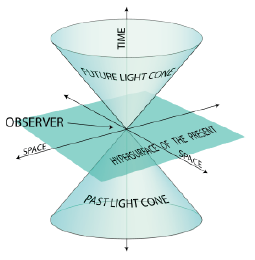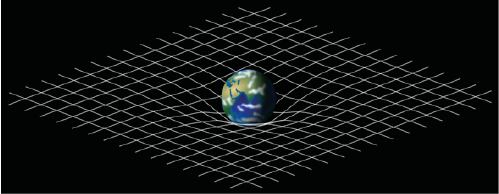## Disproof of Spacetime and General Relativity

Rowland D

Independent Researcher, member of Royal Astronomical Society of Canada and Affiliated by Canadian Association of Physicists

Article
Article Info
Author Info
Figures & Data

## Abstract

Four-dimensional (Minkowski) spacetime is a mathematical fiction that bears no relation to reality. Spacetime does not exist, does not curve, and does not interact with gravity. Because Einstein based his geometric theory of gravity (general relativity) entirely on this false foundation, GR is a fatally flawed theory. This article conclusively disproves both Minkowski spacetime and General Relativity.

## Introduction

In 1916, Albert Einstein published his paper on General Relativity, the geometric theory of gravity . General relativity (GR) states that the force of gravity is associated with the curving/warping of spacetime [2-5]. GR has become the current description of gravity in modern physics. Unfortunately, GR theory suffers from two fatal flaws: (1) as an abstract (nonphysical) form of mathematics, geometry is incapable of interacting with any physical force (e.g., gravity); and (2) spacetime is a mathematical fiction .

In 1905, the same year he received a PhD from the University of Zurich, Einstein developed his famous equation of mass-energy equivalence (E = mc2), which later became known as the theory of special relativity  The only variables in this equation are energy (E), mass (m), and the speed of light (c). Time is not a factor.

In 1908, attempting to better explain his former student’s theory of special relativity (E=mc2), mathematics professor Hermann Minkowski expressed it in terms of a mathematical model that fused time and the three dimensions of space into a single four-dimensional continuum (later known as Minkowski spacetime) . Notice two things about this model:Figure 1: Hypothetical Minkowski 4-D Spacetime

1. It is not possible to assign co-ordinates to the hypothetical time axis; and
2. Because E = mc2 does not include any time variable, there is nowhere said equation fits into this hypothetical four-dimensional model.

## General Relativity

In 1915, Einstein developed his theory of general relativity, the geometric theory of gravitation that is the current description of gravity in modern physics [1, 4]. Einstein proposed that gravity is the result of a geometric distortion of four-dimensional spacetime by massive objects. The more mass that produces gravity in a body, the more distortion you get. This distortion supposedly changes the trajectories of objects moving through space and even the paths of light rays as they pass close by massive objects. Simply stated, massive objects bend the space around them, causing other objects to deviate from the straight lines they otherwise would have followed. Einstein chose the Minkowski spacetime model to depict graphically the gravitational forces supposedly implied in general relativity .Figure 2: Hypothetical Fabric of Spacetime

In this model, a massive object (planet or star) appears to be sitting on a fourth-dimensional spacetime fabric, weighing it down, as a heavy ball would do to a rubber membrane in three dimensions. A beam of light passing close by the Sun, for example, would theoretically follow the lip of the curved spacetime fabric, causing it to bend towards the Sun (rather than pass by it in a straight line). General relativity thus depends on the following three unverifiable assumptions: (1) 4-D spacetime is real, (2) spacetime curves, and (3) spacetime interacts with gravitational forces of massive objects .

#### Spacetime Myth

Spacetime is a mathematical model that supposedly fuses the three dimensions of physical space and the abstract (nonphysical) dimension of time into a single four-dimensional physical continuum. This is a curious graphical excursion that bears no relation to reality.

Suppose a world of two dimensions could exist and you wish to represent it on a three-dimensional graph. How would you know if that circle you see is a sphere, a cone, a cylinder, a dome, or something else? It is not possible to extrapolate meaningful information from two dimensions into three, nor from three into four.

Time measures the changing positions of objects and sequences of events that occur within space. Time is thus an abstract (nonphysical) measurement within the 3-D. Time cannot be extracted from space and projected onto a fourth supposedly physical axis with its own independent set of reference points. Whatever model you create that includes mathematical measurements of an intangible dimension cannot possibly be real. To believe in spacetime is to believe in at least one direction to which one cannot point.

Spacetime cannot curve because spacetime is not real. It is an illusion. All Einstein accomplished with 4-D modelling was a fanciful graphic diversion that cannot possibly exist. Nothing about it explains how gravity could possibly bend light.

#### Disproof of General Relativity

Although general relativity is the accepted definition of gravitation in mainstream physics, this theory is fatally flawed. Spacetime is the geometric illusion that can be expressed algebraically as 3 D + 0 D = 4 D (where D = dimension). Logic tells us that geometric spacetime is not real, does not exist, does not curve, and cannot possibly interact with or be affected by gravity .

Geometry is the mathematics which describes the properties and relations of points, lines, and surfaces – as well as the relative locations of objects. Mathematics is an abstract form of measurement and not a physical thing. As such, geometry can neither cause nor be influenced by anything that exists in physical reality. General relativity fails because it presumes that a physical force (gravity) interacts with an abstraction (geometry) that has no physical existence .

## Conclusions

Four-dimensional spacetime is a mathematical illusion that is hereby disproven. No theory can be valid if it is based on a false assumption. Therefore, Einstein’s theory of General Relativity is also hereby disproven. We are left with the inescapable conclusion that Newton’s universal law of gravitation is the only viable explanation for gravity.

## References

1. Einstein A (1916) Die grundlage der allgemeinen relativitätstheorie. Annalen der Physik 49: 769-822.
2. Einstein A (1905) On the electrodynamics of moving bodies. Annalen der Physik 17: 891.
3. Minkowski Spacetime. Wikipedia.org.
4. General Relativity. Wikipedia.org.
5. Rowland D (2020) What Einstein did not consider about gravity. OSP J Phy Astronomy.

Opinion Article

#### Publication history

Received date: March 05, 2021
Accepted date: March 12, 2021
Published date: March 23, 2021

#### Copyright

©2021 Rowland D. This is an open-access article distributed under the terms of the Creative Commons Attribution License, which permits unrestricted use, distribution, and reproduction in any medium, provided the original author and source are credited.

#### Citation

Rowland D. (2021) Disproof of Spacetime and General Relativity. OSP J Phy Astronomy 2: JPA-2-110

#### David Rowland

Independent Researcher registered with ORCID, Member of Royal Astronomical Society of Canada, and Canadian Association of Physicists
david222@hush.comFigure 1: Hypothetical Minkowski 4-D SpacetimeFigure 2: Hypothetical Fabric of Spacetime